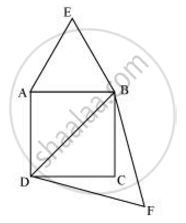# Prove that the area of an equilateral triangle described on one side of a square is equal to half the area of the equilateral triangle described on one of its diagonals - Mathematics

Prove that the area of an equilateral triangle described on one side of a square is equal to half the area of the equilateral triangle described on one of its diagonals

#### SolutionLet ABCD be a square of side a.

Therefore, its diagonal = sqrt2a

Two desired equilateral triangles are formed as ΔABE and ΔDBF.

Side of an equilateral triangle, ΔABE, described on one of its sides = a

Side of an equilateral triangle, ΔDBF, described on one of its diagonals = sqrt2a

We know that equilateral triangles have all its angles as 60º and all its sides of the same length. Therefore, all equilateral triangles are similar to each other. Hence, the ratio between the areas of these triangles will be equal to the square of the ratio between the sides of these triangles.

"Area of Δ ABE"/"Area of ΔDBF"=(a/(sqrt2a))^2

"Area of Δ ABE"/"Area of ΔDBF"=(1/sqrt2)^2=1/2

Concept: Areas of Similar Triangles
Is there an error in this question or solution?

#### APPEARS IN

NCERT Class 10 Maths
Chapter 6 Triangles
Exercise 6.4 | Q 7 | Page 144